## 安装keras

$pip install keras$ pip install tensorflow
\$ python
>>> import os
>>> os.environ['KERAS_BACKENG']='tensorflow'
Using TensorFlow backend.


[TOP]

## 从最小二乘到线性回归

### 最小二乘法

# y = mx + b
# m is slope, b is y-intercept
def compute_error_for_line_given_points(b, m, coordinates):
totalError = 0
for i in range(0, len(coordinates)):
x = coordinates[i]
y = coordinates[i]
totalError += (y - (m * x + b)) ** 2
# example
compute_error_for_line_given_points(1, 2, [[3,6],[6,9],[12,18]])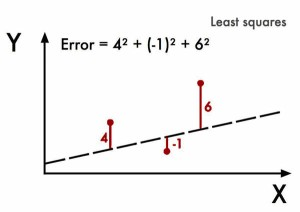Legendre的算法需要反复修改m和b并计算误差平方，非常耗时。

### 梯度下降

1909年，荷兰的Peter Debye简化了Legendre的方法。用Y表示X误差，Legendre的算法是找到使误差最小的X，在下图中，可以看到当X=1.1时，误差Y取到最小值。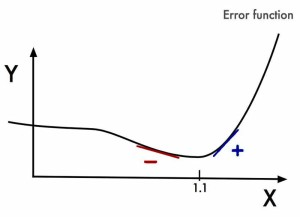Debye注意到最低点左边的斜率是负的，而另一边则是正的。因此，如果知道了任意给定X的斜率值，就可以找到Y的最小值点。这就是梯度下降算法的基本思想，几乎所有的机器学习模型都会用到梯度下降算法。

$Error = x ^5 -2x^3-2$

$Error’ = 5 x ^4 - 6 x^2$

current_x = 0.5 # the algorithm starts at x=0.5
learning_rate = 0.01 # step size multiplier
num_iterations = 60 # the number of times to train the function

#the derivative of the error function (x**4 = the power of 4 or x^4)
def slope_at_given_x_value(x):
return 5 * x**4 - 6 * x**2

# Move X to the right or left depending on the slope of the error function
for i in range(num_iterations):
previous_x = current_x
current_x += -learning_rate * slope_at_given_x_value(previous_x)
print(previous_x)

print("The local minimum occurs at %f" % current_x)


### 线性回归

#Price of wheat/kg and the average price of bread

N = float(len(points))
for i in range(0, len(points)):
x = points[i]
y = points[i]
b_gradient += -(2/N) * (y - ((m_current * x) + b_current))
m_gradient += -(2/N) * x * (y - ((m_current * x) + b_current))
new_b = b_current - (learningRate * b_gradient)
new_m = m_current - (learningRate * m_gradient)
return [new_b, new_m]

def gradient_descent_runner(points, starting_b, starting_m, learning_rate, num_iterations):
b = starting_b
m = starting_m
for i in range(num_iterations):
b, m = step_gradient(b, m, points, learning_rate)
return [b, m]



[TOP]

## 神经网络

### 神经元

1943年，心理学家Warren McCulloch和数学家Walter Pitts参考生物神经元的结构，发表了抽象的M-P神经元模型，并证明了单个神经元可以执行逻辑功能。

1957年，Frank Rosenblatt以M-P模型为基础，提出了感知器(perceptron)模型，并因此登上了《纽约时报》的头条(New Navy Device Learns By Doing)。

from random import choice
from numpy import array, dot, random
result_or = lambda x: 0 if x < 0 else 1
training_data = [ (array([0,0,1]), 0),
(array([0,1,1]), 1),
(array([1,0,1]), 1),
(array([1,1,1]), 1), ]
weights = random.rand(3)
errors = []
learning_rate = 0.2
num_iterations = 100

for i in range(num_iterations):
input, truth = choice(training_data)
result = dot(weights, input)
error = truth - 1_or_0(result)
errors.append(error)
weights += learning_rate * error * input

for x, _ in training_data:
result = dot(x, weights)
print("{}: {} -> {}".format(input[:2], result, result_or(result)))


1969年，MIT AI实验室的Marvin Minsky和Seymour Papert指出感知器无法进行“异或”的运算，还批判了多层感知器构建神经网络模型的想法。尽管此后有人提出新的理论和模型，相关的研究均没有被主流重视，神经网络开始了长达十多年的低潮期。

### 反向传播算法

1986年，D.E.Rumelhart等人在多层神经网络模型的基础上，提出了多层神经网络权值修正的反向传播学习算法——BP算法(Error Back-Propagation)，解决了多层前向神经网络的学习问题，证明了多层神经网络具有很强的学习能力。

$y=f(\omega ^T \cdot x + b)$

$Sigmoid(z)=\frac{1}{1+e^{-z}}$

$TanH(z)=\frac{e^z-e^{-z}}{e^z+e^{-z}}$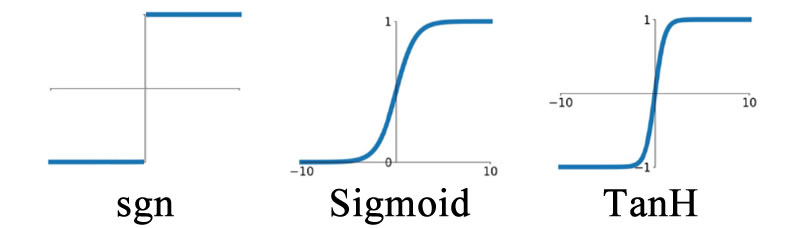$a_1=f(W_1 \cdot x)$

$a_2=f(W_2 \cdot a_1)$

$a_n=f(W_n \cdot a_{n-1})$

$y=f(W_{n+1} \cdot a_n)$

$\omega:=\omega+ \eta x^T e$

$$\eta$$是步长，也被称为学习速率。当达到指定迭代次数或迭代误差时，即可确定$$\omega$$，完成神经元的训练，此时输入一个新的$$x$$，神经元给出一个$$y$$的预测值。

$\delta _i = y_i(1-y_i)(\hat y _i-y_i)$

$\delta _i = a_i(1-a_i)\Sigma \omega _{ki}\delta _k$

$$a_i$$是节点$$i$$的输出值，$$\omega _{ki}$$是节点$$i$$到其下一层节点$$k$$的连接权值，$$\delta _k$$是节点$$i$$的下一层节点$$k$$的误差项。

$\omega _{ji} := \omega _{ji} + \eta \delta _j x _{ji}$

import numpy as np

X_XOR = np.array([[0,0,1], [0,1,1], [1,0,1],[1,1,1]])
y_truth = np.array([,,,])

np.random.seed(1)
syn_0 = 2*np.random.random((3,4)) - 1
syn_1 = 2*np.random.random((4,1)) - 1

def sigmoid(x):
output = 1/(1+np.exp(-x))
return output
def sigmoid_output_to_derivative(output):
return output*(1-output)

for j in range(60000):
layer_1 = sigmoid(np.dot(X_XOR, syn_0))
layer_2 = sigmoid(np.dot(layer_1, syn_1))
error = layer_2 - y_truth
layer_2_delta = error * sigmoid_output_to_derivative(layer_2)
layer_1_error = layer_2_delta.dot(syn_1.T)
layer_1_delta = layer_1_error * sigmoid_output_to_derivative(layer_1)
syn_1 -= layer_1.T.dot(layer_2_delta)
syn_0 -= X_XOR.T.dot(layer_1_delta)

print("Output After Training: \n", layer_2)


### 回归

from keras.models import Sequential
from keras.layers import Dense
import matplotlib as plt


Sequential用于逐层建立神经网络，可以一层一层地添加，Dense表示该神经层为全连接层。

plt.scatter(X,y)
plt.show()


model_r = Sequential()


model_r.add是添加层，此处添加了Dense全连接层，输入和输出数据的维度都是1。

model_r.compile('sgd','mse')


optimizerlosscompile必要的两个参数。keras源码中compile的定义是这样的：

compile(self, optimizer, loss, metrics=None, sample_weight_mode=None)


optimizer： 字符串，指定优化器。下文将会详细讨论优化器的种类、作用和选择方法。

loss： 字符串，损失函数，包括msemaemapemslekldcosine等，关于损失函数的概念，可参考这里，常用的损失函数，可参考这里

metrics： 列表，模型的评估指标，典型用法是metrics=['accuracy']

sample_weight_mode： 样本权重，默认为None，可选temporal，表示按时间步为样本赋权(2D权矩阵)。

model_r.train_on_batch(X_train,y_train)


model_r.evaluate(X_test,y_test,batch_size=40)


model_r.layers.get_weights()


y_pred = model_r.predict(X_test)
plt.scatter(X_test,y_test)
plt.plot(X_test,y_pred)
plt.show()


model_r.save('nn.h5')


from keras.models import load_model


### 分类

from keras.models import Sequential
from keras.layers import Dense, Activation
from keras.optimizers import RMSprop


model_c = Sequential([Dense(32,input_dim=784),Activation('relu'),Dense(10),Activation('softmax'),])


rmsprop = RMSprop(lr=0.001, rho=0.9, epsilon=1e-08, decay=0.0)


model_c.compile(optimizer=rmsprop,loss='categorical_crossentropy',metrics=['accuracy'])


model_c.fit(X_train,y_train,epochs=2,batch_size=32)


model_c.evaluate(X_test,y_test)


[TOP]

## 深度学习

2012年，卷积神经网络(Convolutional Neural Network, CNN)在图像识别领域的突出表现，正式引爆了深度学习的研究。神经网络模型的层数逐年增加，各种优化方法层出不穷。

### ReLU函数

ReLU函数的表达式为：

$ReLU(z)=max(0,z)$

ReLU函数有很多优势，如sigmoid函数需要计算指数和倒数，而ReLU函数则不需要，计算量大幅减小；sigmoid导数最大值为1/4，反向传播算法计算梯度时，每经过一层sigmoid神经元，梯度就要乘上一次sigmoid导数，梯度就会越来越小，对于深度网络就会发生所谓梯度消失的现象，而ReLU导数为1，不会引起梯度变小，因此，使用ReLU激活函数可以训练更深的网络；采用sigmoid激活函数的神经网络，约有50%的神经元会被激活，而ReLU函数在输入小于0时是完全不激活的，因此神经元的激活率更低，节省了计算资源。ReLU有一个缺陷，就是它在负区间的导数为0，可能导致某一个节点永远不参与模型的学习过程。

### Swish函数

$Swish(z)= y = z \cdot sigmoid(z)$

$Swish’(z) = y +sigmoid(z)(1-y)$

### softmax函数

$softmax(z) _i = \frac{e ^{z _i}}{ \Sigma _{k=1} ^{K} e ^{z _{k}}}$

### 损失函数

$f(\omega) = \frac{1}{2}(X \omega -y)^T(X \omega -y)$

$E(\omega) = \frac{1}{2}(X \omega -y)^T(X \omega -y) + \frac{1}{2} \lambda \omega ^T \omega$

$$E(\omega)$$称为误差函数(error function)，$$\lambda$$称为正则化系数，$$\lambda$$越大，正则越强。

• Dropout 在神经网络训练时保持输入和输出层不变，每次迭代过程中“随机”临时删除隐藏层的一部分神经元。采用Dropout的训练过程，相当于训练了很多个只有半数隐藏层神经元的神经网络(“半数网络”)，每一个半数网络都可以给出一个分类结果，这些结果有的是正确的，有的是错误的，随着训练的进行，大部分半数网络都可以给出正确的分类结果，那么少数错误分类结果就不会对最终结果造成大的影响。

• Data Augmentation 即数据扩容，这并不是要真正去重新收集数据以扩充数据集，而是对已有的数据集进行处理，如将图片旋转一个小角度、添加随机噪声、截取图片的一部分等等，增加训练量，从而获得更好的结果。

[TOP]

## 卷积神经网络

CIFAR-10, CIFAR-100

CIFAR是由加拿大先进技术研究院的AlexKrizhevsky, Vinod Nair和Geoffrey Hinton收集的小图片数据集，包含CIFAR-10和CIFAR-100两个数据集。 CIFAR-10由60000张32*32的RGB彩色图片构成，共10个分类。50000张训练，10000张测试（交叉验证）。这个数据集最大的特点在于将识别迁移到了普适物体，而且应用于多分类。CIFAR-100由60000张图像构成，包含100个类别，每个类别600张图像，其中500张用于训练，100张用于测试。其中这100个类别又组成了20个大的类别，每个图像包含小类别和大类别两个标签。官网提供了Matlab,C，python三个版本的数据格式。

• 参数数量太多 考虑一个输入1000*1000像素的图片(一百万像素，现在已经不能算大图了)，输入层有1000*1000=100万节点。假设第一个隐藏层有100个节点(这个数量并不多)，那么仅这一层就有(1000*1000+1)*100=1亿参数，这实在是太多了！我们看到图像只扩大一点，参数数量就会多很多，因此它的扩展性很差。

• 没有利用像素之间的位置信息 对于图像识别任务来说，每个像素和其周围像素的联系是比较紧密的，和离得很远的像素的联系可能就很小了。如果一个神经元和上一层所有神经元相连，那么就相当于对于一个像素来说，把图像的所有像素都等同看待，这不符合前面的假设。当我们完成每个连接权重的学习之后，最终可能会发现，有大量的权重，它们的值都是很小的(也就是这些连接其实无关紧要)。努力学习大量并不重要的权重，这样的学习必将是非常低效的。

• 网络层数限制 我们知道网络层数越多其表达能力越强，但是通过梯度下降方法训练深度全连接神经网络很困难，因为全连接神经网络的梯度很难传递超过3层。因此，我们不可能得到一个很深的全连接神经网络，也就限制了它的能力。

• 局部连接 每个神经元不再和上一层的所有神经元相连，而只和一小部分神经元相连，这样可以减少很多参数。

• 权值共享 一组连接可以共享同一个权重，而不是每个连接有一个不同的权重，这样又减少了很多参数。

• 下采样 使用池化层来减少每层的样本数，进一步减少参数数量，同时还可以提升模型的鲁棒性(Robust，健壮，表征在异常和危险情况下系统的生存能力)。

CNN就是从这些思路出发，尽可能保留重要参数，去掉大量不重要的参数，达到了更好的学习效果。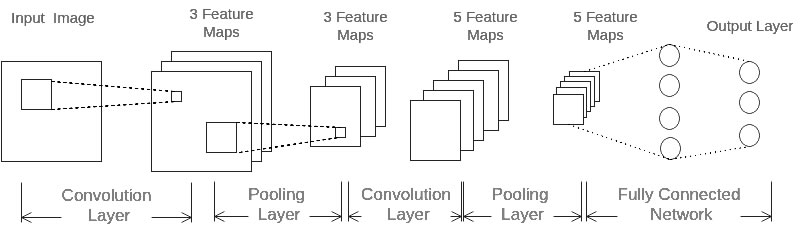CNN每层的神经元都是三维排列的，有长度、宽度和厚度，卷积就是将长宽减小、厚度增加的过程。

### 卷积层的输出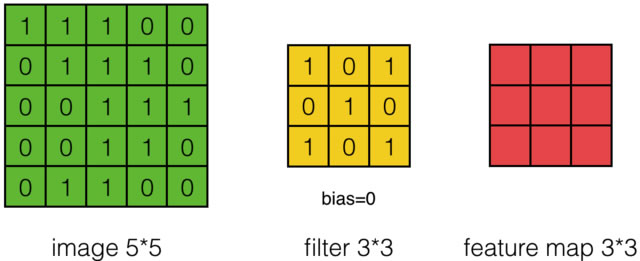$a _{i,j} = f(\Sigma ^2 _{m=0} \Sigma ^2 _{n=0} \omega _{m,n} x _{i+m,j+n} + \omega _b)$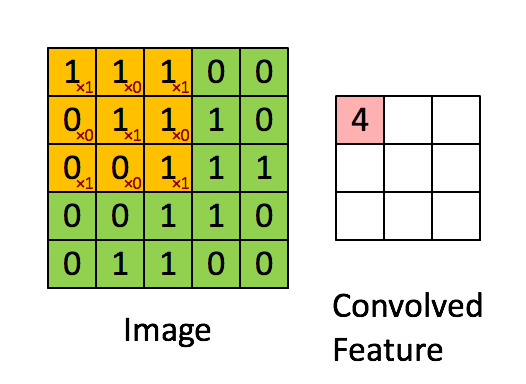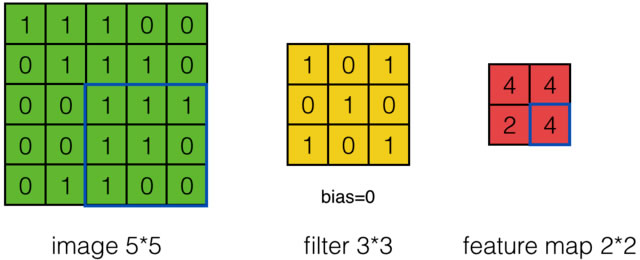$W _2 = (W _1 - F + 2 P)/S +1$

$a _{d,i,j} = f(\Sigma ^{D-1} _{d=0} \Sigma ^{F-1} _{m=0} \Sigma ^{F-1} _{n=0} \omega _{d,m,n} x _{d,i+m,j+n} + \omega _b)$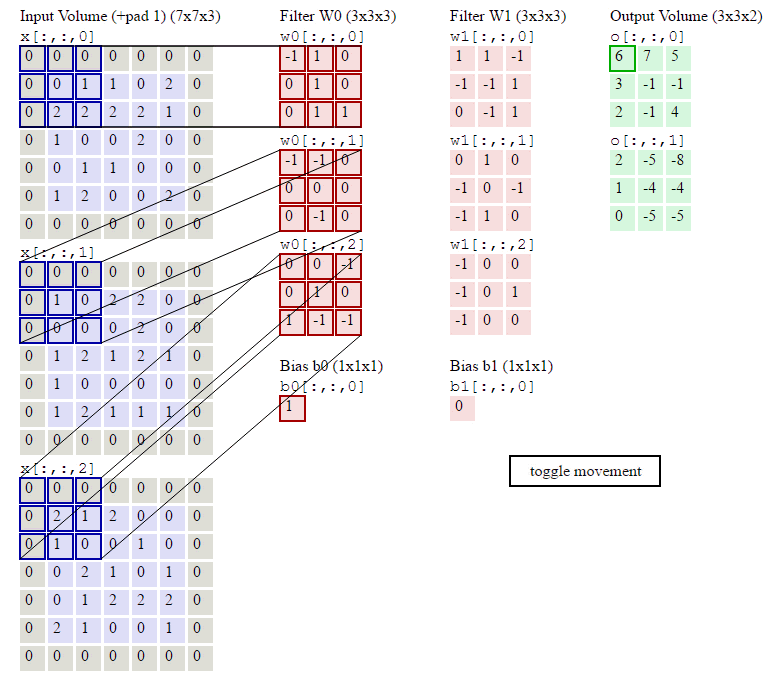### 池化层的输出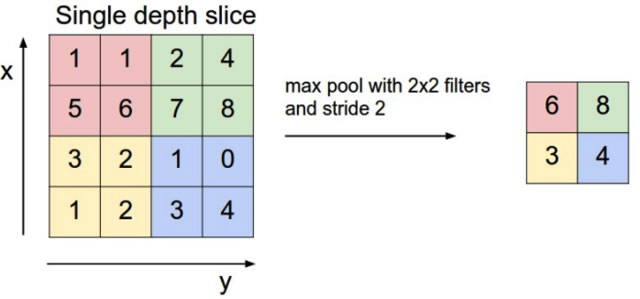CNN的训练采用反向传播算法，首先将误差项从输出层逐层上传，然后计算每个参数的梯度，最后更新参数，详细的推导过程可查看这里

### keras：CNN分类

import numpy as np
from keras.models import Sequential
from keras.layers import Dense, Activation, Convolution2D, MaxPooling2D, Flatten


cnn_c = Sequential()


cnn_c.add(Convolution2D(batch_input_shape=(None,1,28,28),filter=32,kernel_size=5,strides=1,padding='same',data_format='channels_first',))


cnn_c.add(MaxPooling2D(pool_size=2,strides=2,padding='same',data_format='channels_first',))


cnn_c.add(Convolution2D(64,5,strides=1,padding='same',data_format='channels_first'))


cnn_c.add(MaxPooling2D(2,2,'same',data_format='channels_first'))


cnn_c.add(Flatten())


cnn_c.add(Dense(10))


adam = Adam(lr=1e-4)


cnn_c.compile(optimizer=adam,loss='categorical_crossentropy',metrics=['accuracy'])


cnn_c.fit(X_train,y_train,epochs=1,batch_size=64,)


cnn_c.evaluate(X_test, y_test)


[TOP]

## 循环神经网络

RNN最先被用于自然语言处理，比如语言模型就可以使用____来构建。

RNN 最先 被 用于 自然 语言 处理 ， 比如 语言 模型 就 可以 使用 __ 来 构建。

2-Gram模型在填空时，只能看到前面的“使用”，3-Gram可以看到“可以使用”，显然都无法准确完成填空，因为这句话的关键信息“RNN”远在14个词之前。可见N-Gram模型在处理任意长度的句子时并不合适，而且，随着N的增大，模型的复杂度指数级提高，需要占用海量内存。

RNN就为此而生，RNN可以向前或向后看到任意多个词。

### RNN前向计算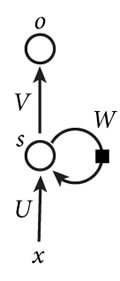$$x$$是输入层的值，$$o$$是输出层的值，$$s$$是隐藏层的值，U是输入层到隐藏层的权重矩阵，V是隐藏层到输出层的权重矩阵，W是隐藏层上一次的值作为这一次的输入的权重矩阵，将该网络在时间维度上展开就得到：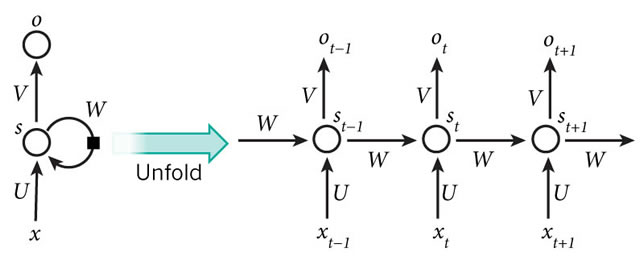$o _t = g(V s _t)$

$s _t = f(U x _t + W s _{t-1})$

$$g$$和$$f$$是激活函数。可以看到$$o _t$$与$$x _t$$、$$x _{t-1}$$、$$x _{t-2}$$、$$x _{t-3}$$、…都有关系，这就是RNN可以向前看任意多输入值的原理。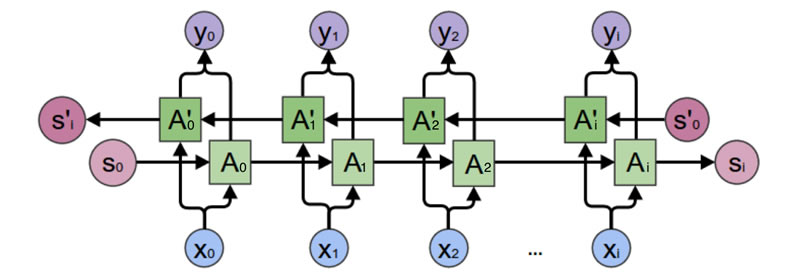$o _t = g(V s _t + V’ s’ _t)$

$s _t = f(U x _t + W s _{t-1})$

$s’ _t = f(U’ x _t + W’ s’ _{t-1})$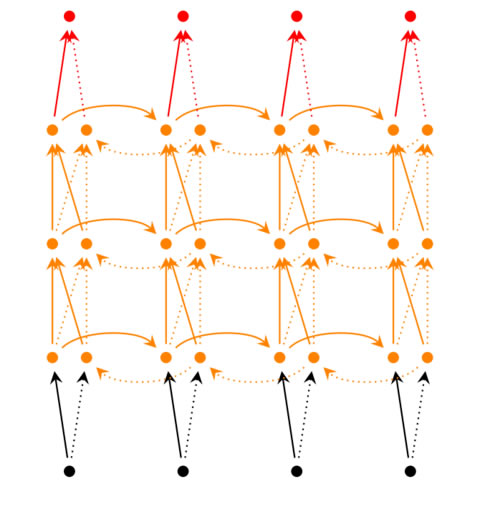$o _t = g(V ^{(i)} s ^{(i)} _t + V’ ^{(i)} s’ ^{(i)} _t)$

$s ^{(i)} _t = f(U ^{(i)} s ^{(i-1)} _t + W ^{(i)} s _{t-1})$

$s’ ^{(i)} _t = f(U’ ^{(i)} s’ ^{(i-1)} _t + W’ ^{(i)} s’ _{t-1})$

$s ^{(1)} _t = f(U ^{(1)} x _t + W ^{(1)} s _{t-1})$

$s’ ^{(1)} _t = f(U’ ^{(1)} x _t + W’ ^{(1)} s’ _{t-1})$

$net _t = U x _t + W s _{t-1}$

$diag(a) = \begin{pmatrix} a _1 & 0 & … 0\\ 0 & a _2 & … 0\\ …\\ 0 & 0 & … a _n \end{pmatrix}$

$\sigma ^T _k = \sigma ^T _t \prod ^ {t-1} _{i=k} W diag [f’(net _i)]$

$(\sigma ^{l-1} _t )^T = (\sigma ^l _t)^T U diag[f’ ^{l-1} (net ^{l-1} _t)]$

$\vert \vert \sigma ^T _k \vert \vert \leqslant \vert \vert \sigma ^T _t \vert \vert \prod ^{t-1} _{i=k} \vert \vert W \vert \vert \vert \vert diag [f’ (net _i)] \vert \vert$

$\leqslant \vert \vert \sigma ^T _t \vert \vert (\beta _W \beta _f) ^{t-k}$

### keras：RNN分类

RNN不只是可以处理语言、视频这样的序列问题，对于与时间无关的问题，也可以按照序列化的方式去处理。

import numpy as np
from keras.models import Sequential
from keras.layers import SimpleRNN, Activation, Dense


TIME_STEPS = 28     # same as the height of the image
INPUT_SIZE = 28     # same as the width of the image
BATCH_SIZE = 50
BATCH_INDEX = 0
OUTPUT_SIZE = 10
CELL_SIZE = 50
LR = 0.001


rnn = Sequential()


rnn.add(SimpleRNN(batch_input_shape=(None, TIME_STEPS, INPUT_SIZE),output_dim=CELL_SIZE,unroll=True))


rnn.add(Dense(OUTPUT_SIZE))


adam = Adam(LR)


rnn.compile(optimizer=adam,loss='categorical_crossentropy',metrics=['accuracy'])


for step in range(4001):
X_batch = X_train[BATCH_INDEX: BATCH_INDEX+BATCH_SIZE, :, :]
Y_batch = y_train[BATCH_INDEX: BATCH_INDEX+BATCH_SIZE, :]
cost = model.train_on_batch(X_batch, Y_batch)
BATCH_INDEX += BATCH_SIZE
BATCH_INDEX = 0 if BATCH_INDEX >= X_train.shape else BATCH_INDEX
if step % 500 == 0:
cost, accuracy = model.evaluate(X_test, y_test, batch_size=y_test.shape, verbose=False)
print('test cost: ', cost, 'test accuracy: ', accuracy)


[TOP]

## 长短时记忆网络

### LSTM前向计算

LSTM引入一个单元状态(cell state)，用来保存长期记忆。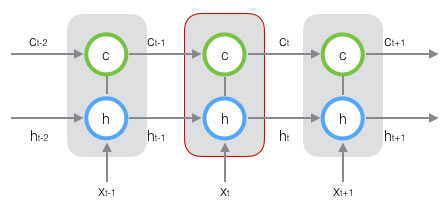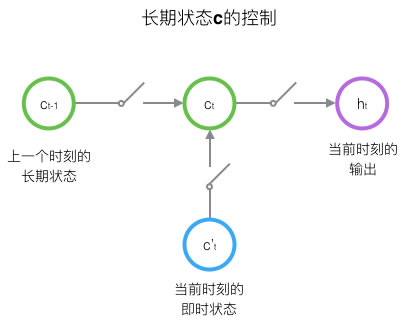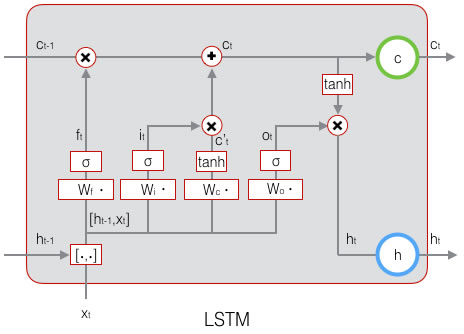$f _t = \sigma (W _f \cdot [h _{h-1} , x _t] + b _f)$

$$W _f$$是遗忘门的权重矩阵，$$[h _{h-1} , x _t]$$表示把这两个向量连接成一个更长的向量，$$b _f$$是遗忘门的偏置项，$$\sigma$$是激活函数。

$[W _f] \begin{pmatrix} h _{h-1}\\ x _t \end{pmatrix} = \begin{pmatrix} W _{fh} & W _{fx} \end{pmatrix} \begin{pmatrix} h _{h-1}\\ x _t \end{pmatrix}$

$W _{fh} h _{h-1} + W _{fx} x _t$

$$W _{fh}$$对应输入项$$h _{t-1}$$，$$W _{fx}$$对应输入项$$x _t$$。

$i _t = \sigma (W _i \cdot [h _{h-1} , x _t] + b _i)$

$$W _i$$是输入门的权重矩阵，$$b _i$$是输入门的偏置项。

$\tilde{c _t} = tanh(W _c \cdot [h _{t-1} , x _t] + b _c)$

$c _t = f _t \circ c _{t-1} + i _t \circ \tilde(c _t)$

$$\circ$$表示按元素乘，即numpy中的’broadcast’。

$o _t = \sigma (W _o \cdot [h _{h-1} , x _t] + b _o)$

LSTM的输出由输出门和单元状态共同确定：

$h _t = o _t \circ tanh(c _t)$

• 遗忘门的权重矩阵$$W _f$$和偏置项$$b _f$$；

• 输入门的权重矩阵$$W _i$$和偏置项$$b _i$$；

• 输出门的权重矩阵$$W _o$$和偏置项$$b _o$$；

• 计算单元状态的权重矩阵$$W _c$$和偏置项$$b _c$$。

LSTM存在相当多的变体，其中GRU(Gated Recurrent Unit)简化了LSTM，仍保持着LSTM的效果，是当前最流行的RNN。

GRU将输入门、遗忘门、输出门变为更新门(update gate)$$z _t$$和重置门(reset gate)$$r _t$$，同时将单元状态和输出合并为一个状态$$h$$，其前向计算公式为：

$z _t = \sigma (W _z \cdot [h _{t-1} , x _t])$

$r _t = \sigma (W _r \cdot [h _{t-1} , x _t])$

$\tilde{h _t} = tanh(W \cdot [r _t \circ h _{t-1} , x _t] )$

$h = (1 - z _t) \circ h _{h-1} + z _t \circ \tilde(h _t)$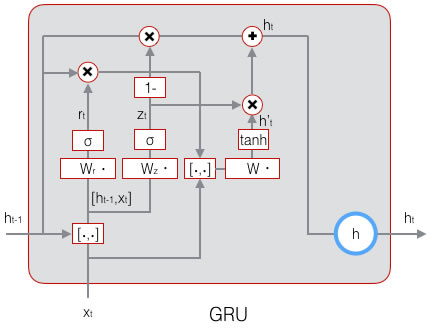### keras：LSTM回归

import numpy as np
from keras.models import Sequential
from keras.layers import LSTM, TimeDistributed, Dense


BATCH_START = 0
TIME_STEPS = 20
BATCH_SIZE = 50
INPUT_SIZE = 1
OUTPUT_SIZE = 1
CELL_SIZE = 20
LR = 0.006


def get_batch():
global BATCH_START, TIME_STEPS
xs = np.arange(BATCH_START, BATCH_START+TIME_STEPS*BATCH_SIZE).reshape((BATCH_SIZE, TIME_STEPS)) / (10*np.pi)
seq = np.sin(xs)
res = np.cos(xs)
BATCH_START += TIME_STEPS
return [seq[:, :, np.newaxis], res[:, :, np.newaxis], xs]


lstm = Sequential()


lstm.add(LSTM(
batch_input_shape=(BATCH_SIZE, TIME_STEPS, INPUT_SIZE),
input_length=TIME_STEPS,
output_dim=CELL_SIZE,
return_sequences=True,
stateful=True
))


lstm.add(TimeDistributed(Dense(OUTPUT_SIZE)))


adam = Adam(LR)


lstm.compile(optimizer=adam,loss='mse')


for step in range(501):
X_batch, Y_batch, xs = get_batch()
cost = model.train_on_batch(X_batch, Y_batch)
pred = model.predict(X_batch, BATCH_SIZE)
plt.plot(xs[0, :], Y_batch.flatten(), 'r', xs[0, :], pred.flatten()[:TIME_STEPS], 'b--')
plt.ylim((-1.2, 1.2))
plt.draw()
plt.pause(0.1)
if step % 10 == 0:
print('train cost: ', cost)


[TOP]